SLUS892D December   2009  – December 2019

PRODUCTION DATA.

1. Features
2. Applications
3. Description
1.     Device Images
4. Revision History
5. Description (continued)
6. Device Comparison Table
7. Pin Configuration and Functions
8. Specifications
9. Detailed Description
1. 9.1 Overview
2. 9.2 Functional Block Diagram
3. 9.3 Feature Description
4. 9.4 Device Functional Modes
10. 10Application and Implementation
1. 10.1 Application Information
2. 10.2 Typical Applications
1. 10.2.1 System with Power Path
2. 10.2.2 Simplified System without Power Path or DPM
3. 10.2.3 Lead-Acid Charging System
11. 11Power Supply Recommendations
12. 12Layout
13. 13Device and Documentation Support
14. 14Mechanical, Packaging, and Orderable Information

• RGE|24
• RGE|24

#### 10.2.1.2.4 Power MOSFETs Selection

Two external N-channel MOSFETs are used for a synchronous switching battery charger. The gate drivers are internally integrated into the IC with 6 V of gate drive voltage. 30-V or higher-voltage rating MOSFETs are preferred for 20-V input voltage and 40-V or higher-rating MOSFETs are preferred for 20-V to 28-V input voltage.

Figure-of-merit (FOM) is usually used for selecting the proper MOSFET based on a tradeoff between the conduction loss and switching loss. For a top-side MOSFET, FOM is defined as the product of the MOSFET ON-resistance, rDS(on), and the gate-to-drain charge, QGD. For a bottom-side MOSFET, FOM is defined as the product of the MOSFET ON-resistance, rDS(on), and the total gate charge, QG.

Equation 17.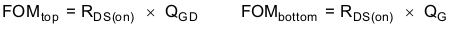The lower the FOM value, the lower the total power loss. Usually lower rDS(on) has higher cost with the same package size.

The top-side MOSFET loss includes conduction loss and switching loss. It is a function of duty cycle (D = VOUT/VIN), charging current (ICHG), the MOSFET ON-resistance tDS(on)), input voltage (VIN), switching frequency (fS), turnon time (ton) and turnoff time (toff):

Equation 18.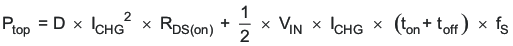The first item represents the conduction loss. Usually MOSFET rDS(on) increases by 50% with 100ºC junction temperature rise. The second term represents the switching loss. The MOSFET turnon and turnoff times are given by:

Equation 19.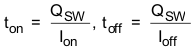where

• Qsw is the switching charge.
• Ion is the turnon gate-driving current.
• Ioff is the turnoff gate driving current.

If the switching charge is not given in the MOSFET data sheet, it can be estimated by gate-to-drain charge (QGD) and gate-to-source charge (QGS):

Equation 20.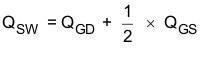Total gate-driving current can be estimated by the REGN voltage (VREGN), MOSFET plateau voltage (Vplt), total turnon gate resistance (Ron), and turnoff gate resistance (Roff) of the gate driver:

Equation 21.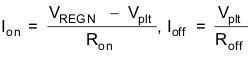The conduction loss of the bottom-side MOSFET is calculated with the following equation when it operates in synchronous CCM:

Equation 22.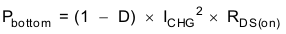If the SRP-SRN voltage decreases below 5 mV (the charger is also forced into nonsynchronous mode when the average SRP-SRN voltage is lower than 1.25 mV), the low-side FET is turned off for the remainder of the switching cycle to prevent negative inductor current.

As a result, all the freewheeling current goes through the body diode of the bottom-side MOSFET. The maximum charging current in nonsynchronous mode can be up to 0.9 A (0.5 A typical) for a 10-mΩ charging-current sensing resistor, considering IC tolerance. Choose the bottom-side MOSFET with either an internal Schottky or body diode capable of carrying the maximum nonsynchronous-mode charging current.

MOSFET gate-driver power loss contributes to the dominant losses on the controller IC when the buck converter is switching. Choosing the MOSFET with a small Qg_total reduces the IC power loss to avoid thermal shutdown.

Equation 23.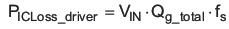where

• Qg_total is the total gate charge for both upper and lower MOSFETs at 6-V VREGN.